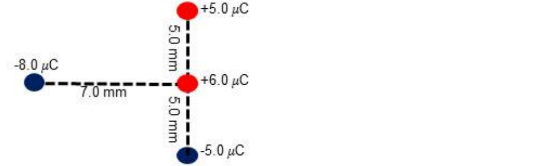Physics Practice Problems Coulomb's Law (Electric Force) Practice Problems Solution: Four charges are arranged as shown in the figure b...

🤓 Based on our data, we think this question is relevant for Professor Moussa's class at UCF.

# Solution: Four charges are arranged as shown in the figure below each with a positive (red) charge or a negative (blue) charge. Find the net total force (magnitude and direction) exerted on the charge with + 6.0 micro coulomb by the other three charges.

###### Problem

Four charges are arranged as shown in the figure below each with a positive (red) charge or a negative (blue) charge. Find the net total force (magnitude and direction) exerted on the charge with + 6.0 micro coulomb by the other three charges.Coulomb's Law (Electric Force)

Coulomb's Law (Electric Force)

#### Q. Two identical small charged spheres hang in equilibrium with equal masses as shown in the figure. The length of the strings are equal and the angle (s...

Solved • Wed Dec 28 2016 10:13:59 GMT-0500 (EST)

Coulomb's Law (Electric Force)

#### Q. A positive test charge q is released near a positive fixed charge Q. As q moves away from Q, it will move with A) increasing acceleration. B) consta...

Solved • Tue Dec 27 2016 15:01:25 GMT-0500 (EST)

Coulomb's Law (Electric Force)

#### Q. A point charge Q = -12 μC, and two other charges, q 1 and q2, are placed as shown. The electric force components on charge Q are Fx = +0.005 N and Fy ...

Solved • Mon Dec 26 2016 14:27:40 GMT-0500 (EST)

Coulomb's Law (Electric Force)

#### Q. Electrical and gravitational forces follow similar equations with one main difference: A) Gravitational forces obey the inverse square law and electr...

Solved • Mon Dec 26 2016 13:17:19 GMT-0500 (EST)